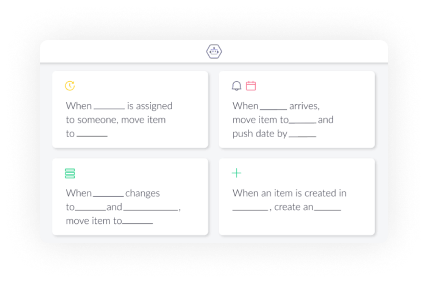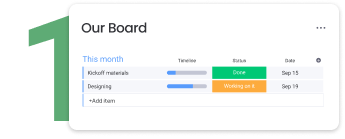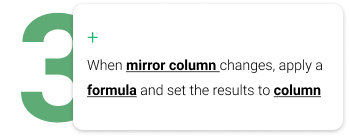<

# How to use Autoboost

to manage your data most efficiently## Enjoy Autoboost in just a few steps## How to useYou should replace them with your columns by clicking the name of the column at the bottom of the formula window.You should replace them with your columns by clicking the name of the column at the bottom of the formula window.

### Check if a value exists

Recipe: when column changes, apply a formula and set result to column.
Formula: IF(HAS_VALUE({item's file}) IS “True“, “Complete“, “Missing file“)
Note: If the output doesn't exists, the output of HAS_VALUE is “False“

### Sum numbers

Recipe: when column changes, apply a formula and set result to column.
Formula: SUM({item's number}, {item's number_1}, 100)
Note: To ensure the result is always updated, add two recipes.
In the trigger - when column changes, choose different columns each time.

### Update item name by status

Recipe: when column changes, apply a formula and set result to item name.
Formula: IF({item’s status} IS “Closed”, CONCATENATE({item's name}, “_”, “closed”),{item's name})

### Concatenate text

Recipe: when column changes, apply a formula and set result to column.
Formula: CONCATENATE({item's people}, ” is the owner of the task”)
Note: You can concatenate every column that can be converted to text.

### Set price by currency

Recipe: when column changes, apply a formula and set result to column.
Formula: IF({item’s currency} IS “USD”, MULTIPLY({item’s cost}, 0.29),
MULTIPLY({item’s cost}, 1))
Note: Update the formula when the currency rate changes.

### Calculate the number of workdays

Recipe: when column changes, apply a formula and set the result to column.
Formula: DAYS(END({item’s timeline}), START({item’s timeline}))

### Copy a mirror column email to text

Recipe: when mirror column changes, apply a formula and set result to column.
Formula: {item's email_mirror}
Note: Set the result to a text column. All the email automation support text columns.

### Assign a person by email

Recipe: when column changes, apply a formula and set result to column.
Formula: {item's email_as_text}
Note: Store the email in a text column, and set the result to a people column.

## How to use subitems values in a formula

1

Write [subitem.column ID] in the formula window.

2

How to find subitems column IDs:

- If this is your first time, activate the developer mode in your monday.com account

(See guidelines below).

- Open your monday.com board in another tab.

- Click the three dots next to the column name.

- Copy the column ID.

3

Open an Autoboost recipe with a formula.
- In the formula window, write [subitem.column ID],
- Replace the "column ID" with the column ID from your board.

## How to activate developer modeChoose monday.labsSearch and activate "Developer mode"Mirror columns can only be copied when they are connected to one board.